### Site Information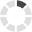Loading... Please wait...

## HVAC News that you don't want to miss.

We constantly publish fresh content about news and products in the HVAC industry. This information is designed to help you, but you'll need to subscribe below!

# How do you calculate the heat (Btu/hr) in an airstream?

Posted on

Air conditioning recirculation is necessary to save energy; however, people need some amount of fresh air in their rooms. Odors and pollutants can be extremely hazardous to one’s health. Taking this into account, a ventilation load from this airflow must be determined for sizing the HVAC equipment. Have you ever wondered how to calculate this load? What components does it have? And, where do the equations found in HVAC manuals or standards come from? This article illustrates how to obtain the equations, the components, and one example which clearly shows the calculation procedure. Have you ever used a psychrometric chart to determine the thermodynamic properties of air? We are going to check how to interpret the input and output data.

In our previous article, we go over the  differences between Sensible Heat and Latent Heat, so let's recap and determine how these contribute to Btu/hr.

## Heat components

### Sensible Heat

When a substance is heated and its temperature increases, this contribution of heat is called sensible heat. Likewise, when heat is extracted from a substance and its temperature drops, this extracted heat is also called sensible heat. In conclusion, it is the heat produced by a temperature change of a substance without phase change. The only device required to measure it is a thermometer.

As promised before, we are going to check step by step the development of the heat relations using the basic thermodynamics principles. Let’s start with the basic heat equation:The mass flow rate is calculated from the ideal gas equation: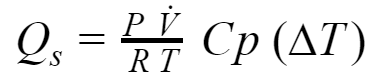Standard conditions are applied for pressure and temperature (found in  Thermodynamics: An Engineering Approach):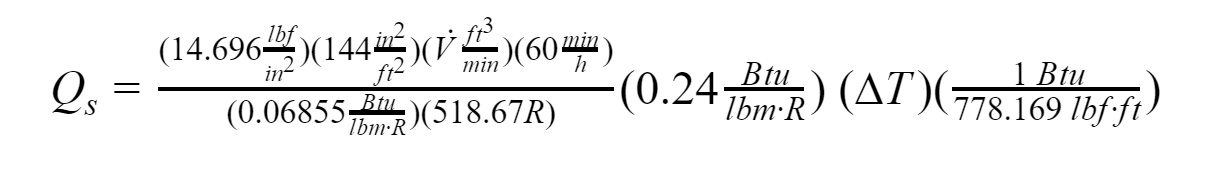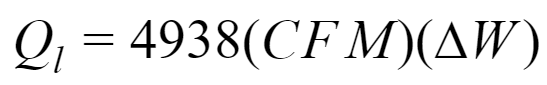Where:

V: Volumetric flow rate, [ft^3/min]

Cp: Specific heat of air, [Btu/lbm.R]

P: Pressure, [psi]

R: Ideal gas constant of air, [Btu/lbm.R]

∆T: Outside/Inside dry bulb temperature Fahrenheit, [°F]

CFM: Infiltration air flow rate

### Latent Heat

It is well known that all substances can change state. Solids become liquids, liquids become gases, etc. The addition or extraction of heat is required to produce these changes. The heat that causes these changes of state without temperature change is called latent heat. It is necessary to use a complex device called a psychrometer, which measures relative humidity.

The initial equation is similar to the previous section:hfg is the latent heat of vaporization. It is defined as the average heat removal required to condense one pound of water vapor from the air within a room. At 14.696, psi is 1076 Btu/lbm, acording to the  Air Conditioning System Design Manual.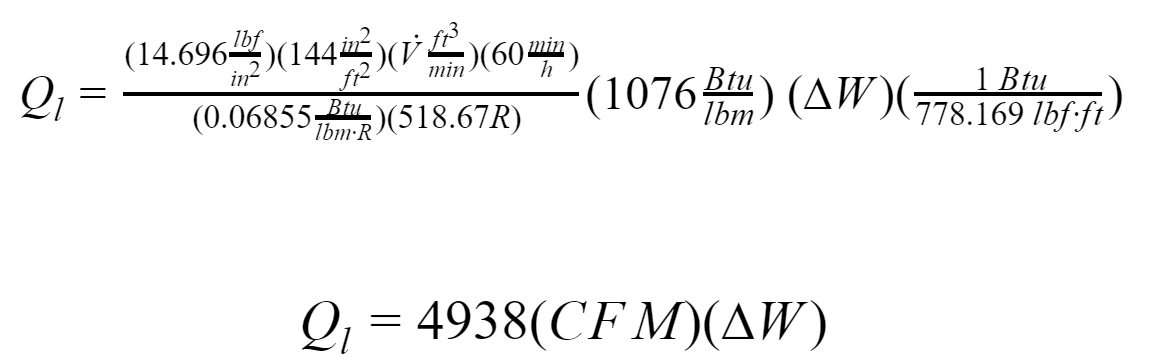Where:

∆W: change in humidity ratio, pound of water per pound of dry air, [lb water/lb dry air]

CFM: Infiltration air flow rate

Other variables are the same stated in sensible heat equation.

### Total Heat

The total heat content of air is the sum of the sensible and latent heat. It is referred to as the enthalpy contained in the air. This thermodynamic property is extremely important, and it is the heat content of air measured in BTU per pound of dry air. The psychrometric chart provides enthalpy data.

The initial equation is similar to the previous sections: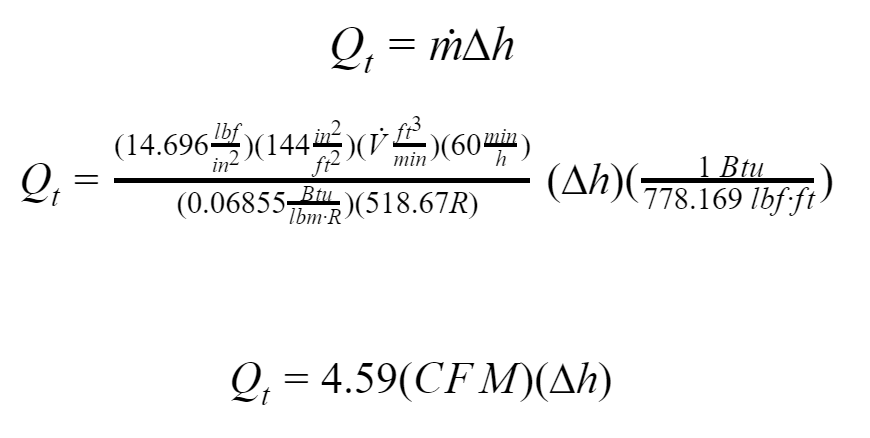Where:

∆h: difference in enthalpy, [Btu/lb]

CFM: Infiltration air flow rate

Now that we understand the parts of calculating the heat (Btu/hr) of an airstream, let's look at an example to apply the calculations.

## Application

The owner of a cafeteria, located at sea level, wants to hire an engineer to size the air conditioning equipment. We are going to determine the total load for ventilation.

Design parameters:

Area = 700 ft^2,

T outside = 88 °F,

T inside = 75 °F.

Relative Humidity = 50%

## Calculation procedure

CFM’s

According the table  6.2.2.1 Minimum Ventilation Rates in the Breathing Zone from ASHRAE Standard 62.1 - Ventilation for Acceptable Indoor Air Quality, the outdoor air rate is 0.18 cfm/ft2; therefore, the total amount of CFM’s is 126.

Sensible Heat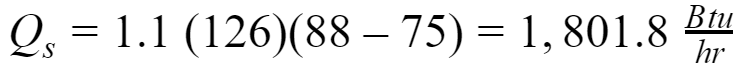Latent Heat

Firstly, it is necessary to find the humidity ratio from the  Psychrometric chart. Do you understand clearly how to interpret the air properties in the psychrometric chart? You may not be familiar with this chart; however, it is necessary that you know how to get each property for each point. Figure 1 illustrates the indoor and outdoor state points. We have inserted black boxes to let you know where are the labels and the curves that you need to follow to get the psychrometric properties. The corresponding values are presented in Table 1. You know that practice makes the master, so I invite you to read Chapter 1: Psychrometrics of the ASHRAE Handbook - Fundamentals to understand deeply those concepts.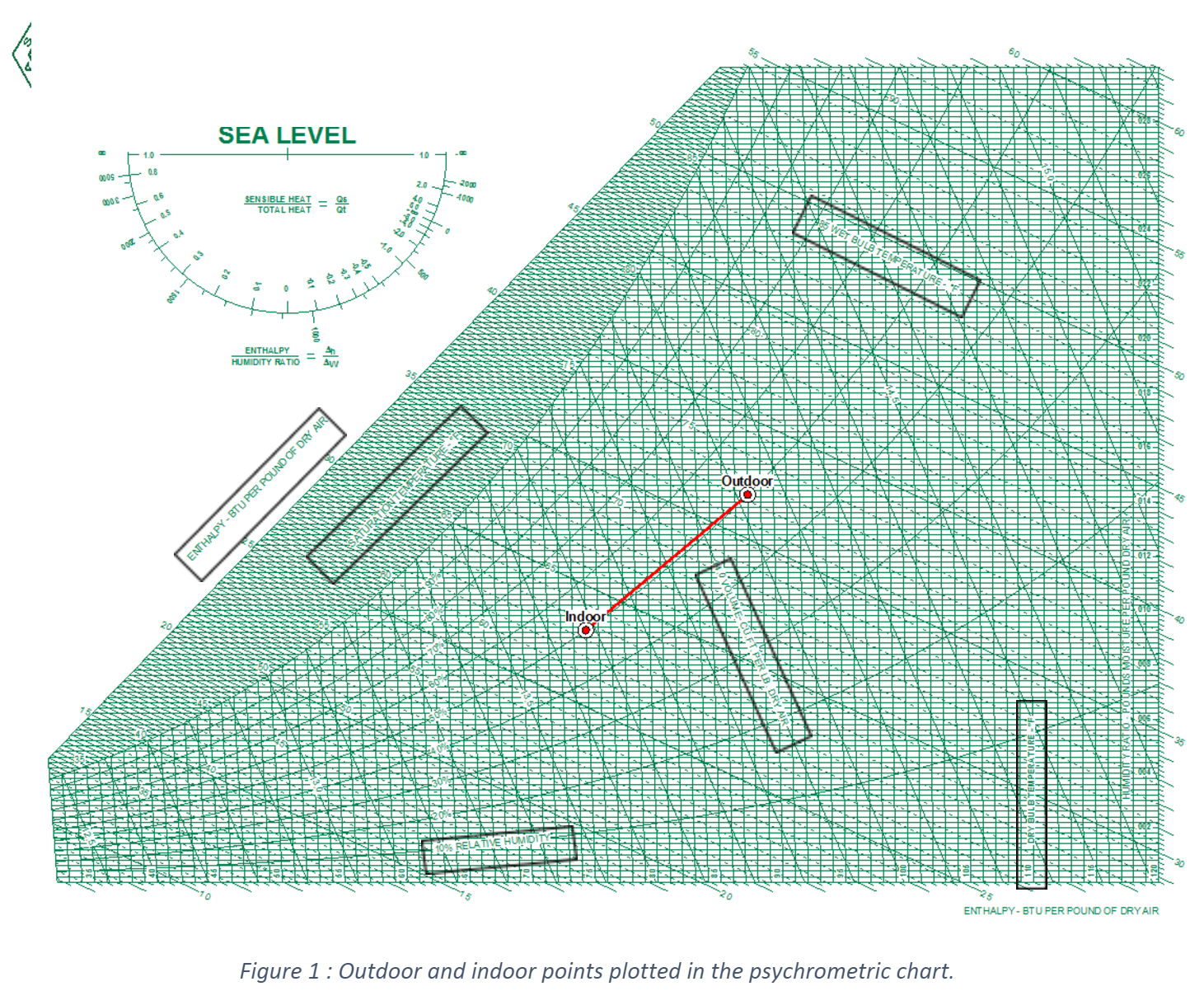Table 1 : Psychrometric properties of air at indoor and outdoor conditions at sea level.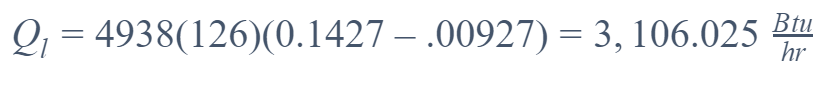Total Heat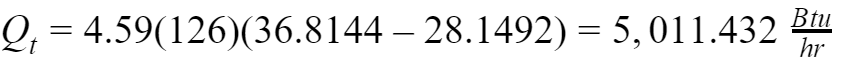Also, we can derive the total heat as the sum of the sensible and latent heat.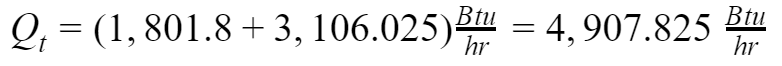Figure 2 shows the calculations from a  Psychrometric Chart Software. In general, a software tool has more precision and accuracy because it is possible to perform several iterations.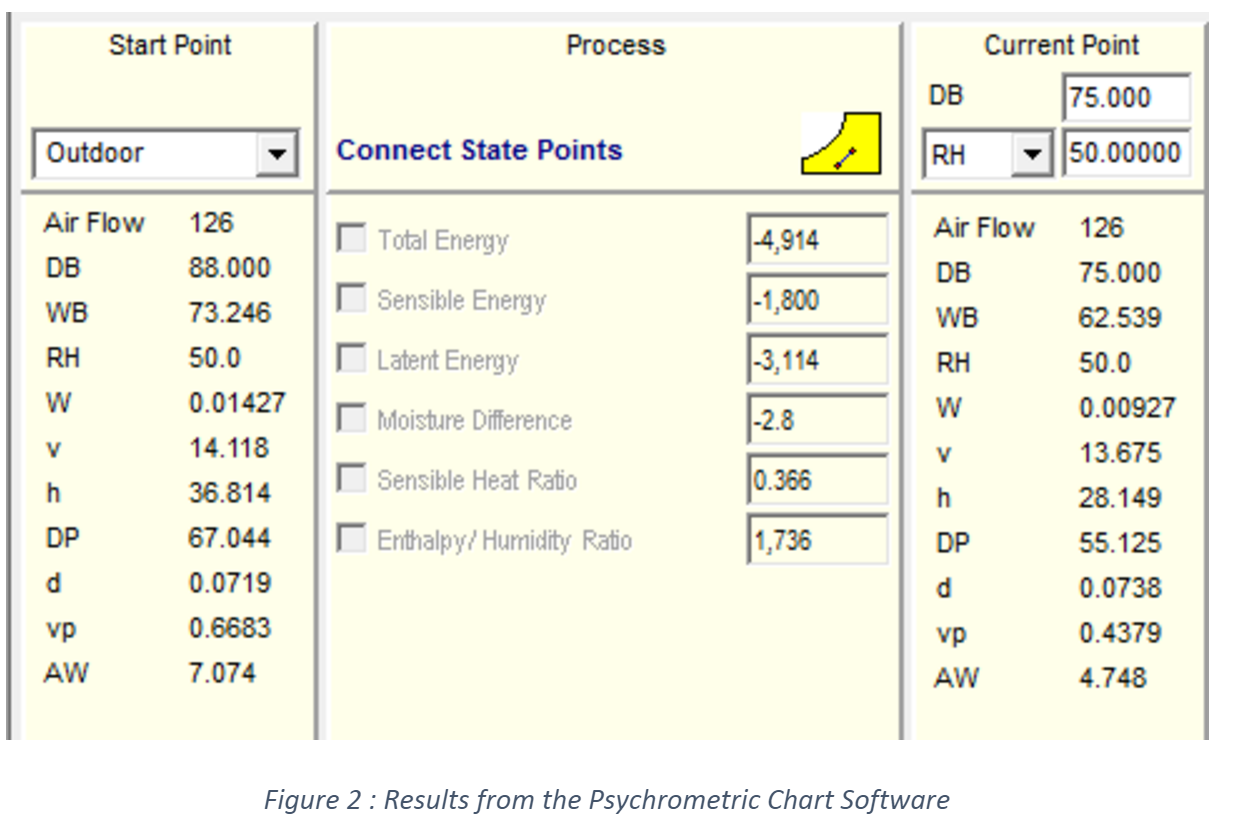Error calculation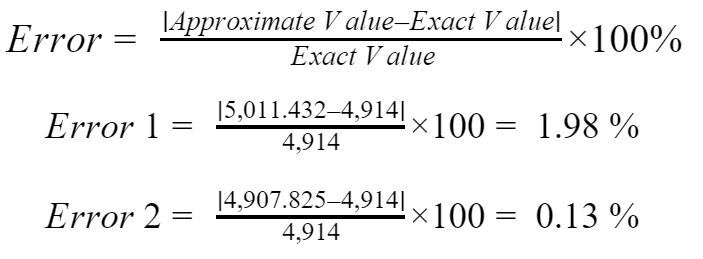## Conclusions

1. This calculation procedure is applicable to any airstream.
2. The equations described here are found in different HVAC standards and manuals. It is important to mention that these equations are approximations without greatly compromising accuracy. If exact calculations are required, properties in each point must be determined according to the height above sea level.
3. The HVAC equipment sizing depends on a variety of loads (number of people, equipment, heat by radiation and conduction, ventilation, etcetera). In some cases, the ventilation load is around 50 % of the total load. HVAC engineers cannot omit this parameter.
4. The errors of 1.98 % and 0.13 % found previously states that the presented equations for QS and QL have considered average thermodynamic properties like Cp and hfg. Those errors are negligible; therefore, the equations can be used without any concern.